## Do you also find mathematics and calculus annoying and difficult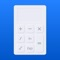# Scientific Calculus Calculator

by APPSACE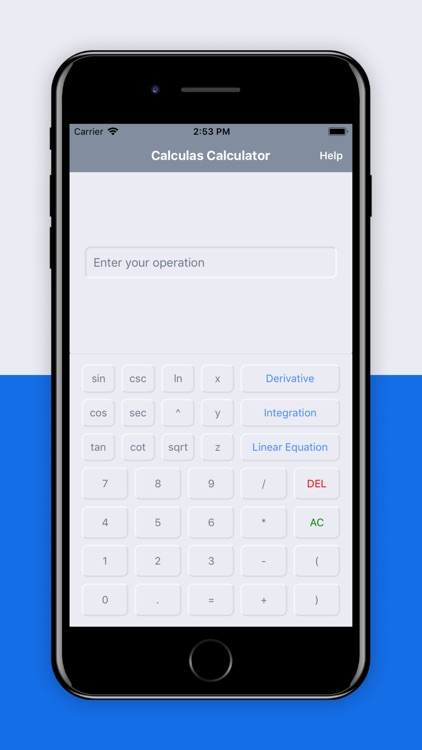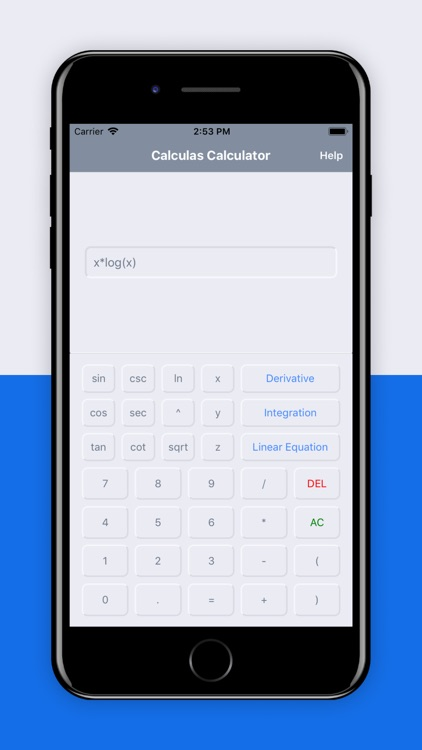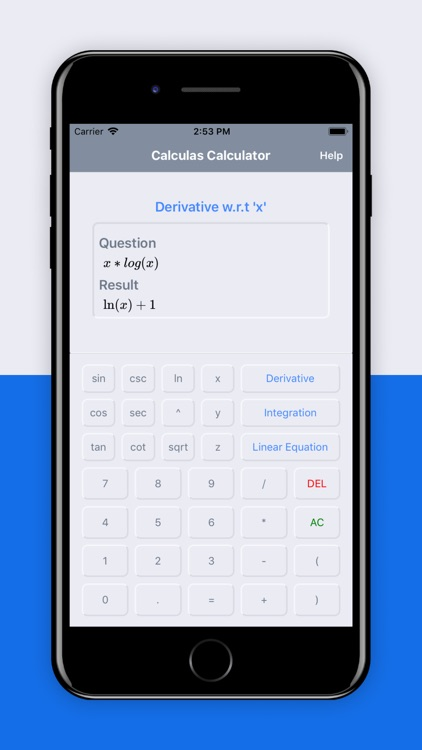Do you also find mathematics and calculus annoying and difficult? Never mind, Scientific Calculus Calculator is here to help you improve your mathematical skills. Scientific Calculus Calculator can come up with instant solutions for all your Math problems. Whether you are facing difficulties in simple linear equations or integrals, two or three variable equations, or calculating derivatives, Scientific Calculus Calculator has you covered. It is no less than a blessing for matric students, college students as well as for FSc students. All you have to do is just enter the derivations, equations, or integrations carefully and let this calculator do the rest.### App Details

Version
1.0
Rating
NA
Size
13Mb
Genre
Education Utilities
Last updated
April 23, 2020
Release date
April 23, 2020

### App Screenshots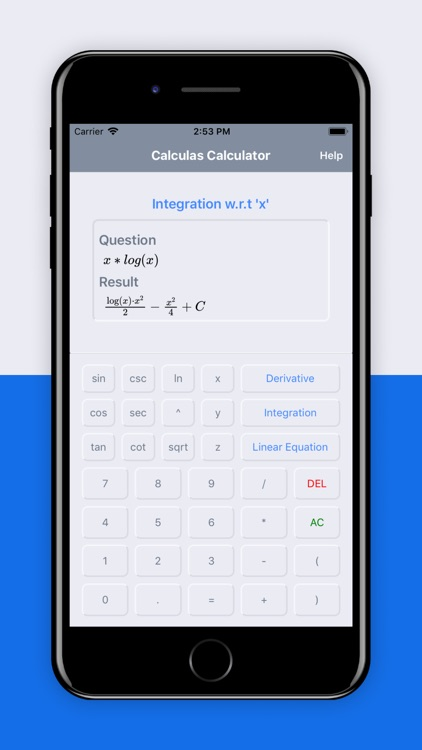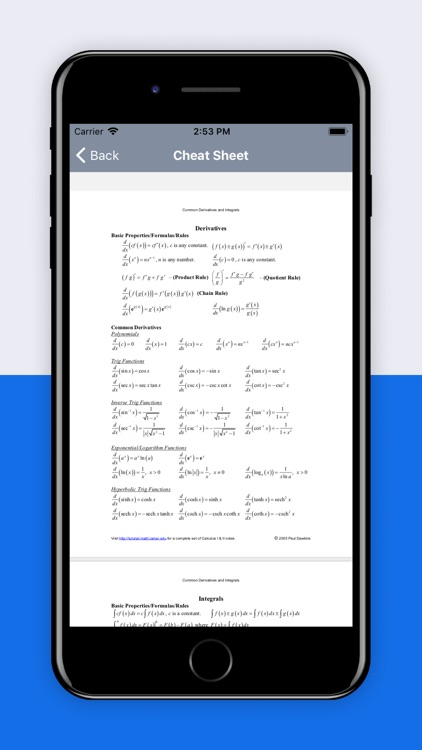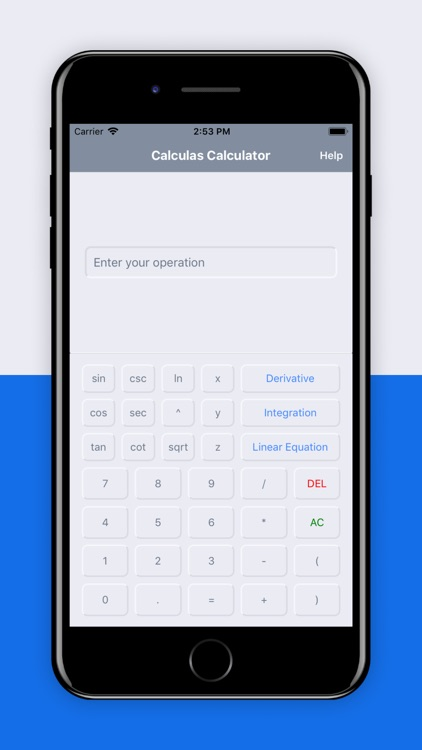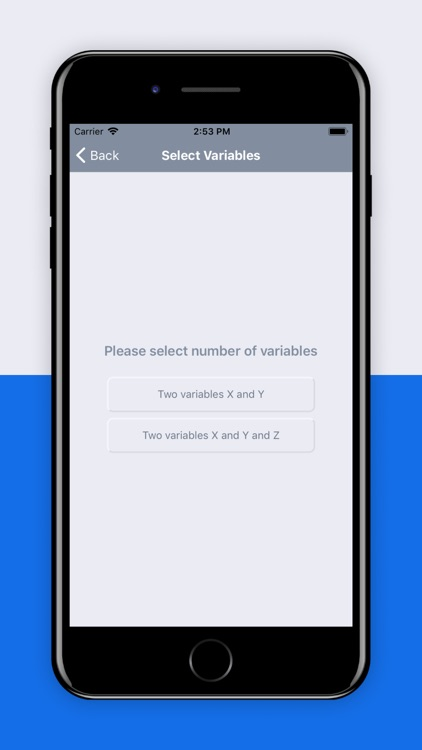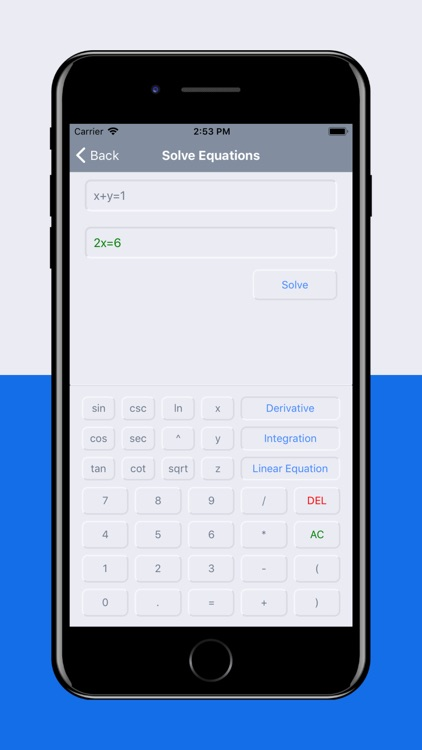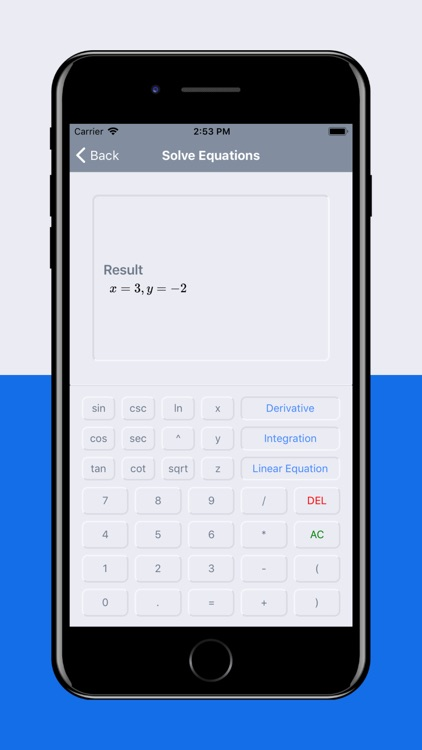### App Store Description

Do you also find mathematics and calculus annoying and difficult? Never mind, Scientific Calculus Calculator is here to help you improve your mathematical skills. Scientific Calculus Calculator can come up with instant solutions for all your Math problems. Whether you are facing difficulties in simple linear equations or integrals, two or three variable equations, or calculating derivatives, Scientific Calculus Calculator has you covered. It is no less than a blessing for matric students, college students as well as for FSc students. All you have to do is just enter the derivations, equations, or integrations carefully and let this calculator do the rest.
Scientific Calculus Calculator - A Derivative Calculator
With the help of Scientific Calculus Calculator, you can calculate the derivations online for free. Just type any function, and you will find the solutions along with the step-by-step method and graph. Calculating derivatives will now be as easy as ABC, and soon you will be able to solve derivatives of other usual functions at your fingertips.
Scientific Calculus Calculator - An Integral Calculator
Solving definite and indefinite integrals is not that difficult with Scientific Calculus Calculator. This online calculator is not only an integral solver, but it allows you to calculate antiderivative, improper, and double, and triple derivatives. A step-by-step solution and graph are also included in the process. Could there be anything better than this for college and matric students? Practice and prepare for your exams without any extra class or tuitions with the help of the step-by-step guide.
Scientific Calculus Calculator - A Linear Equation Solver
This online tool also allows you to solve the algebraic equations as well. Now solve linear equations with one variable, two variables, or three variables. Attempting algebraic problems will now be easier than ever. Enhance your mathematical skills with this multiple equation solver.
Other Features of Scientific Calculus Calculator
• Backspace
The backspace option allows users to correct any typing error or mistake instead of erasing all the values and starting all over again.
• Memory
The calculator stores the equations or numbers you entered, so you have the option to continue later from where you left.
• Convenient Layout
The app is quite easy to use. Users can see the values and calculations clearly since every digit on the screen is easy to read.
Promotional Statement
Make your smartphones and other Android devices more useful by downloading our fast, safe, and free app. Scientific Calculus Calculator helps in making subjects like Math and calculus easier and fun for all students and allows them to make the best possible use of their cell phones for educational purposes. Download the app now and take a step towards smart learning.

Disclaimer:
AppAdvice does not own this application and only provides images and links contained in the iTunes Search API, to help our users find the best apps to download. If you are the developer of this app and would like your information removed, please send a request to takedown@appadvice.com and your information will be removed.